# NCERT Class 10: Science-Exercise Solutions

### Chapter 10-Light-Reflection and Refraction-Exercise Solutions

NCERT Book Exercise –Page Number 185-186

Q.1. Which one of the following materials cannot be used to make a lens ?
(a) Water
(b) Glass
(c) Plastic
(d) Clay

Ans. (d) Clay

Q.2. The image formed by a concave mirror is observed to be virtual, erect and larger than the ob¬ject. Where should be the position of the object ?
(a) Between the principal focus and the centre of curvature
(b) At the centre of curvature
(c) Beyond the centre of curvature
(d) Between the pole of the mirror and its principal focus.

Ans. (d) Between the pole of the mirror and its principal focus.
Q.3. Where should an object be placed in front of a convex lens to get a real image of same size as the object?
(a) At the principal focus of the lens
(b) At twice the focal length
(c) At infinity
(d) Between the optical centre of the lens and its principal focus

Ans. (b) At twice the focal length .
Q.4. A spherical mirror and a thin spherical lens each have focal length of –15 cm. The mirror and the lens are likely to be :
(a) both concave
(b) both convex
(c) the mirror is concave and the lens is convex
(d) the mirror is convex, but the lens is concave

Ans. (a) Both concave
Q.5. No matter how far you stand from a mirror, your image appears erect. The mirror is likely to be:
(a) plane
(b) concave
(c) convex
(d) either plane or convex

Ans. (d) Either plane or convex
Q.6. Which of the following lenses would you prefer to use while reading small letters found in a dictionary ?
(a) A convex lens of focal length 50 cm
(b) A concave lens of focal length 50 cm
(c) A convex lens of focal length 5 cm
(d) A concave lens of focal length 5 cm

Ans. (c) A convex lens of focal length 5 cm
Q.7. We wish to obtain an erect image of an object, using a concave mirror of focal length 15 cm. What should be the range of distance of the object from the mirror? What is the nature of the image? Is the image larger or smaller than the object? Draw a ray diagram to show the image formation in this case.

Ans. Range of the distance of the object = within 15 cm from the pole of the mirror.
Nature of the image = virtual, erect and larger than the object.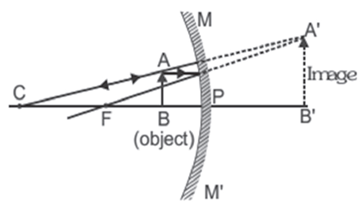Q.8. Name the type of mirror used in the following situations:
(b) Side/rear-view mirror of a vehicle
(c) Solar furnace

Ans. (a) Concave Mirror: This is because a concave mirror can produce powerful parallel beam of light when the light source is placed at its principal focus.
(b) Convex Mirror: This is because of its wider field of view.
(c) Concave Mirror: This is because it concentrates the parallel rays of sun at principal focus.
Q.9. One-half of a convex lens is covered with a black paper. Will this lens produce a complete image of the object? Verify your answer experimentally. Explain your observations.
Ans. The convex lens will form complete image of an object, even if its one half is covered with black paper. It can be understood by the following two cases :

Case I : When the upper half of the lens is covered :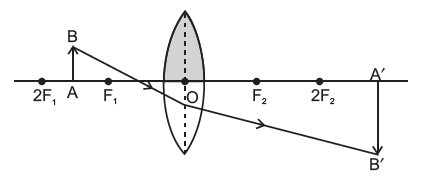In this case, a ray of light coming from the object will be refracted by the lower half of the lens. These rays meet at the other side of the lens to form the image of the given object, as shown in the above figure.
Case II. When the lower half of the lens is covered:

In this case, a ray of light coming from the object is refracted by the upper half of the lens. These rays meet on the other side of the lens to form the image of the given object, as shown in the figure below.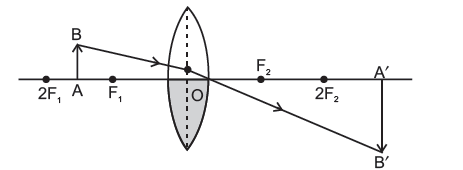Q.10. An object 5 cm in length is held 25 cm away from a converging lens of focal length 10 cm. Draw the ray diagram and find the position, size and the nature of the image formed.
Ans. Height of the object, h0= 5 cm
Distance of the object from converging lens, u = – 25 cm
Focal length of converging lens, f = 10 cm
Using lens formula,
1/v – 1/u = 1/f
1/v = 1/f + 1/u = = 1/10 – 1/25 =3/ 50 =16.66 cm
Also, for a converging lens, hi/ho=v/u
hi = v/u × ho =(50  5) /( 3  (- 25)) = 10/(-3)= –3.3 cm
Thus, the image is inverted and formed at a distance of 16.7 cm behind the lens and measures 3.3 cm. The ray diagram is shown below: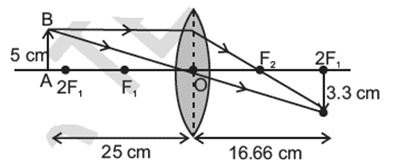Q.11. A concave lens of focal length 15 cm forms an image 10 cm from the lens. How far is the object placed from the lens? Draw the ray diagram.

Ans. Focal length of concave lens (OF1), f = – 15 cm
Image distance, v = – 10 cm
According to the lens formula,
1/v – 1/u = 1/f
1/u = 1 /v -1/f =
1/ u = 1/(-10) – 1/(-15) =(-1)/10 + 1/15 = -1/30
u = – 30 cm
The negative value of u indicates that the object is placed 30 cm in front of the lens. This is shown in the following ray diagram.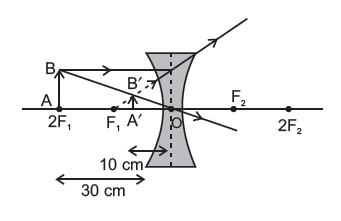Q.12. An object is placed at a distance of 10 cm from a convex mirror of focal length 15 cm. Find the position and nature of the image.

Ans. Focal length of convex mirror, f = +15 cm
Object distance, u = – 10 cm
According to the mirror formula,
1/v + 1/u = 1/f
1/v = 1/f- 1/u = 1 /15 + 1/10 = 5/30
v= = 6 cm
The positive value of v indicates that the image is formed behind the mirror.
Magnification, m = Image Distance /Object Distance =- v/u = -6 /-10 = + 0.6
The positive value of magnification indicates that the image formed is virtual and erect.
Q.13. The magnification produced by a plane mirror is +1. What does this mean ?

Ans. The positive sign means image formed by a plane mirror is virtual and erect. Since the magnification is 1 it means that the size of the image is equal to the size of the object.
Q.14. An object 5 cm is placed at a distance of 20 cm in front of a convex mirror of radius of curvature 30 cm. Find the position, nature and size of the image.

Ans. Object distance, u = – 20 cm
Object height, h = 5 cm
Radius of curvature, R = 30 cm
Radius of curvature = 2 × Focal length
R = 2f
f = 15 cm
According to the mirror formula,
1/v + 1/u = 1/f
1/v = 1/f -1/u = 1/15 + 1/ 20 = (4 + 3)/ 60 = 7/60
v= 60/7
v = 8.57 cm
The positive value of v indicates that the image is formed behind the mirror.
Magnification, m = Image Distance /Object Distance
= −8 57/20 = 0.428
The positive value magnification indicates that the image formed is virtual.
Magnification, m = Height of the Image/ Height of the Object = hi/h0
h′ = m × h = 0.425 × 5 = 2.14 cm
The positive value of image height indicates that the image formed is erect.
Therefore, the image formed is virtual, erect, and smaller in size.
Q.15. An object of size 7.0 cm is placed at 27 cm in front of a concave mirror of focal length 18 cm. At what distance from the mirror should a screen be placed, so that a sharp focussed image can be obtained ? Find the size and the nature of the image.

Ans. Object distance, u = – 27 cm
Object height, h = 7 cm
Focal length, f = – 18 cm
According to the mirror formula,
1/v + 1/u =1/f
1/v = 1/f – 1/u = -1/18 + 1/27
v = – 54 cm
The screen should be placed at a distance of 54 cm in front of the given mirror.
Magnification, m = Image Distance /Object Distance = −54/27 = – 2
The negative value of magnification indicates that the image formed is real.
Magnification, m = Height of the Image/Height of the Object = hi/h0
hi = 7 × (– 2) = – 14 cm
The negative value of image height indicates that the image formed is inverted.

Q.16. Find the focal length of a lens of power –2.0 D. What type of lens is this?
Ans. Power of lens, P = 1/f
P = – 2 D
f = – 1/2 = – 0.5 m
A concave lens has a negative focal length. Hence, it is a concave lens.

Q.17. A doctor has prescribed a corrective lens of power +1.5 D. Find the focal length of the lens. Is the prescribed lens diverging or converging?
Ans. Power of lens, P =1/f
P = 1.5 D
f = 1/1.5 = 10/15= 0.66 m .
A convex lens has a positive focal length. Hence, it is a convex lens or a converging lens.